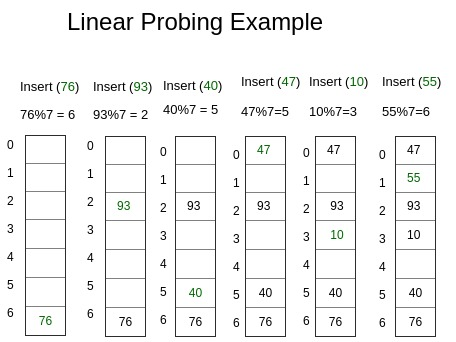Open in App
Not now

# Implementing own Hash Table with Open Addressing Linear Probing

• Difficulty Level : Medium
• Last Updated : 26 Dec, 2022

Prerequisite – Hashing Introduction, Implementing our Own Hash Table with Separate Chaining in Java
In Open Addressing, all elements are stored in the hash table itself. So at any point, size of table must be greater than or equal to total number of keys (Note that we can increase table size by copying old data if needed).

• Insert(k) – Keep probing until an empty slot is found. Once an empty slot is found, insert k.
• Search(k) – Keep probing until slot’s key doesn’t become equal to k or an empty slot is reached.
• Delete(k) – Delete operation is interesting. If we simply delete a key, then search may fail. So slots of deleted keys are marked specially as “deleted”.

Here, to mark a node deleted we have used dummy node with key and value -1.
Insert can insert an item in a deleted slot, but search doesn’t stop at a deleted slot.
The entire process ensures that for any key, we get an integer position within the size of the Hash Table to insert the corresponding value.
So the process is simple, user gives a (key, value) pair set as input and based on the value generated by hash function an index is generated to where the value corresponding to the particular key is stored. So whenever we need to fetch a value corresponding to a key that is just O(1).Implementation:

## CPP

 `#include ``using` `namespace` `std;` `// template for generic type``template` `<``typename` `K, ``typename` `V>` `// Hashnode class``class` `HashNode {``public``:``    ``V value;``    ``K key;` `    ``// Constructor of hashnode``    ``HashNode(K key, V value)``    ``{``        ``this``->value = value;``        ``this``->key = key;``    ``}``};` `// template for generic type``template` `<``typename` `K, ``typename` `V>` `// Our own Hashmap class``class` `HashMap {``    ``// hash element array``    ``HashNode** arr;``    ``int` `capacity;``    ``// current size``    ``int` `size;``    ``// dummy node``    ``HashNode* dummy;` `public``:``    ``HashMap()``    ``{``        ``// Initial capacity of hash array``        ``capacity = 20;``        ``size = 0;``        ``arr = ``new` `HashNode*[capacity];` `        ``// Initialise all elements of array as NULL``        ``for` `(``int` `i = 0; i < capacity; i++)``            ``arr[i] = NULL;` `        ``// dummy node with value and key -1``        ``dummy = ``new` `HashNode(-1, -1);``    ``}``    ``// This implements hash function to find index``    ``// for a key``    ``int` `hashCode(K key)``    ``{``        ``return` `key % capacity;``    ``}` `    ``// Function to add key value pair``    ``void` `insertNode(K key, V value)``    ``{``        ``HashNode* temp = ``new` `HashNode(key, value);` `        ``// Apply hash function to find index for given key``        ``int` `hashIndex = hashCode(key);` `        ``// find next free space``        ``while` `(arr[hashIndex] != NULL``               ``&& arr[hashIndex]->key != key``               ``&& arr[hashIndex]->key != -1) {``            ``hashIndex++;``            ``hashIndex %= capacity;``        ``}` `        ``// if new node to be inserted``        ``// increase the current size``        ``if` `(arr[hashIndex] == NULL``            ``|| arr[hashIndex]->key == -1)``            ``size++;``        ``arr[hashIndex] = temp;``    ``}` `    ``// Function to delete a key value pair``    ``V deleteNode(``int` `key)``    ``{``        ``// Apply hash function``        ``// to find index for given key``        ``int` `hashIndex = hashCode(key);` `        ``// finding the node with given key``        ``while` `(arr[hashIndex] != NULL) {``            ``// if node found``            ``if` `(arr[hashIndex]->key == key) {``                ``HashNode* temp = arr[hashIndex];` `                ``// Insert dummy node here for further use``                ``arr[hashIndex] = dummy;` `                ``// Reduce size``                ``size--;``                ``return` `temp->value;``            ``}``            ``hashIndex++;``            ``hashIndex %= capacity;``        ``}` `        ``// If not found return null``        ``return` `NULL;``    ``}` `    ``// Function to search the value for a given key``    ``V get(``int` `key)``    ``{``        ``// Apply hash function to find index for given key``        ``int` `hashIndex = hashCode(key);``        ``int` `counter = 0;` `        ``// finding the node with given key``        ``while` `(arr[hashIndex] != NULL) { ``// int counter =0; // BUG!` `            ``if` `(counter++ > capacity) ``// to avoid infinite loop``                ``return` `NULL;` `            ``// if node found return its value``            ``if` `(arr[hashIndex]->key == key)``                ``return` `arr[hashIndex]->value;``            ``hashIndex++;``            ``hashIndex %= capacity;``        ``}` `        ``// If not found return null``        ``return` `NULL;``    ``}` `    ``// Return current size``    ``int` `sizeofMap()``    ``{``        ``return` `size;``    ``}` `    ``// Return true if size is 0``    ``bool` `isEmpty()``    ``{``        ``return` `size == 0;``    ``}` `    ``// Function to display the stored key value pairs``    ``void` `display()``    ``{``        ``for` `(``int` `i = 0; i < capacity; i++) {``            ``if` `(arr[i] != NULL && arr[i]->key != -1)``                ``cout << ``"key = "` `<< arr[i]->key``                     ``<< ``"  value = "``                     ``<< arr[i]->value << endl;``        ``}``    ``}``};` `// Driver method to test map class``int` `main()``{``    ``HashMap<``int``, ``int``>* h = ``new` `HashMap<``int``, ``int``>;``    ``h->insertNode(1, 1);``    ``h->insertNode(2, 2);``    ``h->insertNode(2, 3);``    ``h->display();``    ``cout << h->sizeofMap() << endl;``    ``cout << h->deleteNode(2) << endl;``    ``cout << h->sizeofMap() << endl;``    ``cout << h->isEmpty() << endl;``    ``cout << h->get(2);` `    ``return` `0;``}`

Output

```key = 1  value = 1
key = 2  value = 3
2
3
1
0
0```

Complexity analysis for Insertion:

• Time Complexity:
• Best Case: O(1)
• Worst Case: O(N). This happens when all elements have collided and we need to insert the last element by checking free space one by one.
• Average Case: O(1) for good hash function, O(N) for bad hash function
• Auxiliary Space: O(1)

Complexity analysis for Deletion:

• Time Complexity:
• Best Case: O(1)
• Worst Case: O(N)
• Average Case: O(1) for good hash function; O(N) for bad hash function
• Auxiliary Space: O(1)

Complexity analysis for Searching:

• Time Complexity:
• Best Case: O(1)
• Worst Case: O(N)
• Average Case: O(1) for good hash function; O(N) for bad hash function
• Auxiliary Space: O(1) for search operation

This article is contributed by Chhavi. If you like GeeksforGeeks and would like to contribute, you can also write an article using write.geeksforgeeks.org or mail your article to review-team@geeksforgeeks.org. See your article appearing on the GeeksforGeeks main page and help other Geeks.